×
##### Chapter Notes

1General Physiology
CHAPTERS
CHAPTERS
• 1. Body Fluid: Distribution and Measurement
• 2. Cell Membrane, Transport and Membrane Potential2

# Body Fluid: Distribution and MeasurementChapter 1

BODY COMPOSITION (AS % OF BODY WEIGHT)
Human body is composed of the following:
Atomic/Elementary level
Molecular or, chemical level
Histology/Tissue level
Oxygen
60%
Water
60%
Skeletal muscle
36%
Carbon
20%
Protein
18%
Non-skeletal
29%
Hydrogen
15%
Fat
15%
25%
Calcium
1%
Mineral
6%
Bone
10%
Others
4%
Glycogen
1%

Body Water as % Body Weight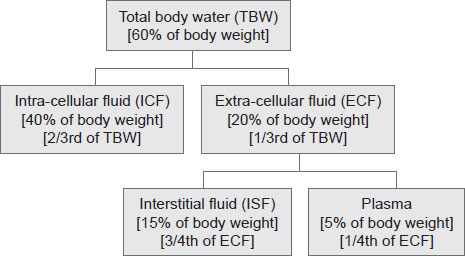• Total blood volume = 8% of body weight (Since, plasma volume = 5% of body weight and blood cell volume = 3% of body weight)
Total blood volume: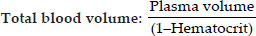Transcellular Fluid
This compartment includes fluid in the synovial, peritoneal, pericardial, and intraocular spaces, as well 4as the cerebrospinal fluid; it is usually considered to be a specialized type of ECF, although in some cases, its composition may differ markedly from that of the plasma or ISF. All the transcellular fluids together constitute about 1 to 2 liters (1% to 3% of body weight).
• Pericardial fluid: 50 ml
• Pleural fluid: 10–20 ml
• Peritoneal fluid: Male–no fluid, Female–20 ml (early luteal phase) and 5 ml (late luteal phase).
• Synovial fluid:
• About 1 ml of fluid is present in each large joint like knee, hip, ankle, shoulder, elbow and wrist (range 0.5 ml to 1.5 ml). In Osteoarthritis it may be upto 100 ml.
Mesenchymal tissue fluid: Fluid in the mesenchymal tissues such as dense connective tissue, cartilage and bones contain about 6% of the body water. The interstitial fluid, transcellular fluid and mesenchymal tissue fluid combinedly form the 75% of ECF.
Some Important Aspects of Body Fluid:
• Due to relatively greater amount of adipose tissue in the females, the TBW is ~10% less in a normal young adult female than that in an average adult male. In both sexes the value decreases with age.
• Brain (contains 74% to 80% of water) forms a large fraction and bones (contains 20% water) form a small fraction of body weight in infant as compared to adult. Because of this infant contains proportionately more body fluid than adult.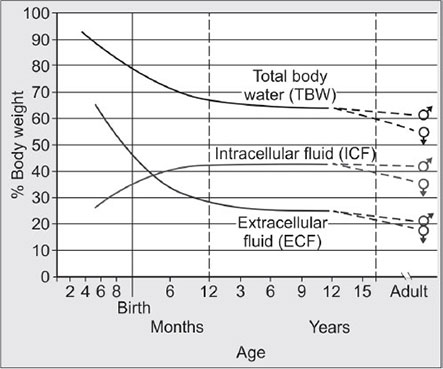Fig. 1: Body fluid (as percentage of body) changes with age
5
• In infant relatively more fluid is present in ECF compartment (prone to dehydration). Thus, the ECF to ICF fluid volume ratio is larger in infants and children as compared to adults.
• The normal postnatal diuresis causes an immediate decrease in the ECF volume. It is followed by continued expansion of the ICF volume, which results from cellular growth.
• By the age of 3–4 month postnatal life ICF and ECF volume becomes equal. And by 1 year of age, the ratio of the ICF volume to the ECF volume approaches adult levels.
• By the age of two years, total body fluid percentage approaches the adult percentage of 60% and at puberty body fluid percentage and distribution becomes equal to adult percentage.
• At puberty, for the first time the sex difference in fluid content appears.
• Total body water volume in different age group is given in the following table:
Age
~ Total body water (As % of body weight)
Premature infant
90%
Full term Newborn
70-80%
One year
64%
Puberty to 39 years
Men: 64%
Women: 52%
40 to 60 years
Men: 55%
Women: 47%
>60 years
Men: 52%
Women: 46%
Ref: Fluid and Electrolyte Balance By Norma M. Metheny

Measurement of Body Fluid
The volume of water in different compartment can be measured by Volume of distribution principle (indicator dilution principle).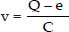Where,ν = Volume of fluidQ = Quantity of indicator givenC = Concentration of the indicatore = The amount of indicator which has either been lost or metabolized
6

Substances Used for Measurement of Various Compartments
Compartment
Indicator used
Plasma volume
Evans’ blue (T1824), 125I-albumin (most commonly used)
RBC volume
Tagging RBC with 51Cr, 59Fe, 32P; also antigenic tagging
Total blood volume
Plasma volume/(1–Hematocrit)
ECF volume
Inulin, sucrose, Mannitol, Sodium thiosulphate, sodium thiocyanate, 22Na, 125I-iothalamate
Interstitial fluid
Cannot be measured directly; can be calculated as (ECF volume–Plasma volume)
ICF
Cannot be measured directly; can be calculated as (Total body water–ECF)
Total body water
D2O is most frequent used; also, tritium oxide, antipyrine

Normal Fluid Balance in Body
• Daily intake of water: Total 2300 ml (Fluids ingested 2100 ml and from metabolism 200 ml)
• Daily loss of water:
• Insensible Water Loss 700 ml (from skin 350 ml and from lungs 350 ml)
• Sweat 100 ml
• Feces 100 ml
• Urine 1400 ml
• Minimum water requirement in adult is 1.5 L/day

Shifts of Water between Compartments
It is important to understand how volume and osmolality of ECF and ICF in abnormal states changes. The relationship is easily explained by Darrow-Yannet Diagram, which is explained below: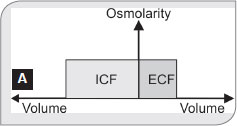• Remember:
• Water moves rapidly across cell membranes; therefore, the osmolarities of ICF and ECF remain almost exactly equal to each other except for a few minutes after a change in one of the compartments.
• Cell membranes are almost completely impermeable to many solutes; therefore, the number of osmoles in the ECF or ICF generally remains constant unless solutes are added to or lost from the ECF compartment.7
• Y-axis: solute concentration or, osmolality
• X-axis: Volume of ICF (2/3) and ECF (1/3)
• In normal control state, osmolality of ICF and ECF is equal.
• Whenever any solution is added or, lost from body, its changes the ECF volume or, osmolality or, both according to the type of solution (isotonic, hypotonic or, hypertonic).
• ICF volume varies with ECF osmolality and ICF osmolality changes inversely to volume change.

Now Carefully Check the Given Diagrams
(Broken line indicates shifts from normal).

Gain of Isotonic Fluid (B)
Infusion of isotonic solution will lead to increase ECF volume but no change in osmolality. Because there is no change in osmolality, ICF volume remains same.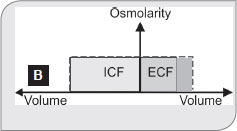Loss of Isotonic Fluid (C)
Will only decrease the ECF volume.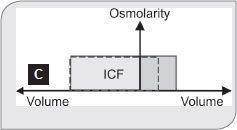Gain of Hypotonic Fluid (D)
It increases the ECF volume, but because of its hypotonicity, the final osmolality of ECF decreases. Water shifts from ECF to ICF (osmosis), which leads to increase ICF volume. Because its only shift of water from ECF to ICF, osmolality of ICF reduces until new equilibrium reached.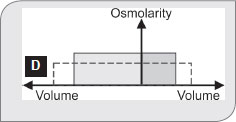Loss of Hypotonic Fluid (E)
Hypotonic loss leads to decrease volume but increase osmolality of ECF. Water shifts from ICF to ECF (osmosis), which decrease ICF volume.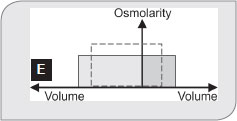Gain of Hypertonic Fluid (F)
Increase in effective volume and osmolality of ECF. Water shifts from ICF to ECF, which leads to decrease ICF volume and increase osmolality until new equilibrium reached.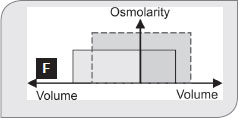Loss of Hypertonic Fluid (G)
Decrease ECF volume and tonicity because of hypertonic fluid loss. Decrease tonicity of ECF, shifts the fluid from ECF to ICF (osmosis). So, ICF volume increases and tonicity decreases.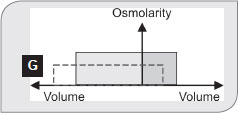8

SUMMARY OF DARROW-YANNET DIAGRAM
Type (diagram no)
Example
ECF volume
ICF volume
ECF osmolarity
Gain of isotonic fluid (B)
Isotonic NaCl infusion
Increased
No change
No change
Loss of isotonic fluid (C)
Hemorrhage
Diarrhea
Vomiting
Decreased
No change
No change
Gain of hypotonic fluid (D)
Drinking of tap water
Increased
Increased
Decreased
Loss of hypotonic fluid (E)
Sweating,
Diabetes insipidus
Decreased
Decreased
Increased
Gain of Hypertonic fluid (F)
Excessive NaCl intake, mannitol
Increased
Decreased
Increased
Loss of Hypertonic fluid (G)
Decreased
Increased
Decreased

COMPOSITION OF DIFFERENT FLUID COMPARTMENT

ECF
Ionic composition of plasma and interstitial fluid (ISF) is similar.
• The most important difference between these two compartments is the higher concentration of protein in the plasma.
• Because of the Donnan effect, negatively charge plasma protein holds a slightly greater (~2%) cations in the plasma than in the interstitial fluid.

ICF
Only small quantities of Na+ and Cl ions and almost no Ca++ ions. Instead, it contains large amounts of K+ and phosphate ions plus moderate quantities of Mg++ and sulfate ions. Also, cells contain large amounts of protein, almost four times as much as in the plasma.
9

TYPICAL IONIC CONCENTRATION OF MAMMALIAN CELL
Ion
Cell (ICF) in mOsm/L
ISF in mOsm/L
Blood (ECF) in mOsm/L
K+
140 (max cation)
4
4.2
Na+
14
139
145 (max cation)
Cl
4
108
108 (max anion)
HCO3
10
28
24
HPO4, H2PO4
11 (max anion)
2
2
Protein
4
0.2
1.2
Ca2+
<0.0002
1.2
1.8
Mg2+
20
0.7
1.5
Corrected osmolar activity (mOsm/L)
281
281
282
Total osmotic pressure at 37°C (mm Hg)
5423
5423
5443

UNITS OF MEASUREMENTS

Moles
A mole is the molecular weight of the substance in grams. Each mole (mol) consists of 6 × 1023 molecules. 10The millimole (mmol) is 1/1000 of a mole, and the micromole (μmol) is 1/1,000,000 of a mole.
Example: 1 mol of NaCl = 23 g + 35.5 g = 58.5 g, and 1 mmol = 58.5 mg. (Atomic weight of Na = 23 and CL = 35.5)

EQUIVALENTS
One equivalent (Eq) is 1 mol of an ionized substance divided by its valence.
Examples: One mole of NaCl dissociates into1Eq of Na+ and 1 Eq of Cl. One equivalent of Na+ = 23 g, but 1 Eeq of Ca2+ = 40 g/2 = 20 g.

OSMOLE
It expresses concentration of osmotically active particles.
1 Osmole = Mol/Number of freely moving particular each molecule liberates in solution
Examples:
• 1 mol of NaCl = 2 osmoles because each NaCl molecule given one Na+ and one Cl particle is solution.
• 1 mol of Na2SO4 = 3 osmoles because each Na2SO4 molecule gives 2 Na+ and 1 SO4 in solution
• 1 mol of, CaCl2 = 3 osmoles, because each molecule of CaCl2 give 3 particles (1 calcium and 2 Cl) in solution.

CONVERTING WEIGHT TO OSMOLALITY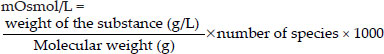CONVERTING WEIGHT TO MILLIEQUIVALENTS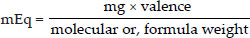Example A: Convert the expression 10 mg% of Ca++ to mEq/L and mOsmol/L
1. 10 mg% of Ca++ means, 10 mg/100 ml= 100 mg/L
2. According to the above equation: mEq/L =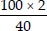= 5 mEq/L11
3. According to equation: mOsmol/L =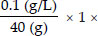1000 = 2.5 mOsmol/L
Example B: How many mEq of KCl are in 1.5 g of KCl
1. Molecular weight of KCl = 39 + 35.5 = 74.5
2. According to equation: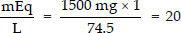mEq/L
Example C: 100 mg each of NaCl, CaCl2 and FeCl3 in 1 L of water. Determine the concentration in percent, molarity, milliequivalent (mEq) and milliosmolarity (mOsmol).
Percent (g/volume)
• NaCl: 100 mg of NaCl in 1 L solution = 0.1 g/1000 ml = 0.01 g/100 ml = 0.01%
• CaCl2: 100 mg of CaCl2 in 1 L solution = 0.1 g/1000 ml = 0.01 g/100 ml = 0.01%
• FeCl3: 100 mg of FeCl3 in 1 L solution =0.1 g/1000 ml = 0.01 g/100 ml = 0.01%
• Molarity NaCl: Molecular weight = 58.5 g (so, 1 Mol = 58.5 g) 0.01 g/58.5 g = 0.0017 M = 1.7 mMol
• CaCl2: Molecular weight = 111 g (so, 1 Mol = 111 g) 0.01 g/111 g = 0.0009 M = 0.9 mMol
• FeCl3: Molecular weight = 162.2 g (so, 1 Mol = 162.2 g) 0.01 g/162.2 g = 0.00062 M = 0.62 mMol
Milliequivalent
• NaCl: Molecular weight = 58.5 g valence 1
• CaCl2: Molecular weight = 111 g and valence 2
• FeCl3: Molecular weight = 162.2 g and valence 3
So according to formula: mEq =12

MILLIOSMOLE
We have already discussed, that 1 mol of NaCl = 2 osmoles, 1 mol of, CaCl2 = 3 osmoles. Similarly 1 mol FeCl3 = 4 osmoles.
So,
1.7 mM of NaCl = (2 × 1.7) mOsmol = 3.4 mOsmol/L
0.9 mM of CaCl2 =(3 × 0.9) mOsmol = 2.7 mOsmol/L
0.62 mM of FeCl3 = (4 × 0.62) = 24.8 mOsmol/L
Or, directly using the formula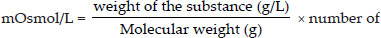species × 1000 will give the same result.

TONICITY
Definition: This is the osmolality of a solution with respect to plasma osmolality.
Example: 0.9% NaCl is isotonic; 5% glucose is isotonic initially; later has become hypotonic.

Calculated Osmolarity (CO)/Osmolality
When unit of Na and K are in mEq/L or, mmol/L, and unit of Glucose and BUN are in mg/dl
13
When all units are in mmol/L:

Measured Osmolality (MO)
The most accurate way of finding out the osmolality is by freezing point depression.

OSMOLAL GAP
If there is a difference between the CO and MO, it is called osmolal gap. Osmolol gap indicates the presence of a foreign substance like usually a toxic alcohol such as ethanol, methanol or isopropyl alcohol.
So, sometime a different equation is used when the patient has ingested ethanol, or, mannitol therapy:

TONICITY OF DIFFERENT SOLUTION
Tonicity
mOsmol/Kg
Glucose (g/L)
Dextrose solution
5%
Isotonic but physiologically hypotonic
278
50
Provide 170 Cal/L
10%
Hypertonic
556
100
Provide 340 Cal/L
Saline solution
0.45%
Hypotonic
154
0
No Calories
0.9%
Isotonic
308
0
Only solution that may be administered with blood product
3%
Hypertonic
1026
0
No Calories14
Dextrose in saline
5% in 0.225%
Isotonic
355
50
Provide 170 Cal/L
5% in 0.45%
Hypertonic
432
50
Provide 170 Cal/L
5% in 0.9%
Hypertonic
586
50
Provide 170 Cal/L
Multiple electrolytes solution
Ringer's solution
Isotonic
309
0
Similar in composition to plasma except it has excess Cl and No Mg++, no HCO3
Lactate Ringer's (Hartmann's Solution)
Isotonic
274
0
Similar in composition to plasma except it has No Mg++
15
MULTIPLE CHOICE QUESTIONS
BODY FLUIDS

RECENT MCQs
1. Volume of ICF in body:
1. 0.2 × body wt.
2. 0.4 × body wt
3. 0.6 × body wt.
4. 0.8 × body wt.
2. ECF is how much part of total body weight:
1. One third
2. Half
3. Two third
4. None
3. ICF is:
1. 14 L
2. 20% of body weight
3. 28 L
4. 33% of body weight
4. Major cation in ECF:
1. Na+
2. K+
3. Ca++
4. Mg++
5. Most osmotically active intracellular cation:
1. K+
2. Na+
3. Mg+2
4. Protein
6. True regarding Na+ ion:
1. Responsible for Donnan effect
2. Responsible for Resting membrane potential
3. Responsible for Depolarization
4. Does not help other ions in trans port
7. Water content of lean body mass is (in ml/100 gm of tissue):
1. 40
2. 50
3. 60
4. 70
8. Exchangeable potassium in the body is:
1. 10%
2. 40%
3. 70%
4. Almost 100%
9. The normal plasma oncotic pressure is:
1. 10 mm Hg
2. 15 mm Hg
3. 20 mm Hg
4. 30 mm Hg
10. All are seen more in ECF except:
1. Na+
2. Cl
3. Mg++
4. HCO3
11. Daily water loss in sweat during normal activities:
1. 50-100 ml
2. 200-400 ml
3. 500-700 ml
4. 1000-1200 ml
12. The majority of body sodium is present:
1. ECF
2. ICF
3. Plasma
4. Bone
13. Bound potassium is mainly found in the following except:
1. Brain
2. Bones
3. RBC's
4. Platelets
14. Volume of blood in a child, per kg of body weight:
1. 70-80
2. 100-150
3. 150-200
4. 200-250
15. Plasma protein contributes only ~1 mosm/litre in plasma osmolality because of:
1. High concentration, Low molecular weight
2. Low concentration, High molecular weight
3. Low concentration, Low molecular weight
4. High concentration, High molecular weight16
16. Plasma protein contributes only ~1 mosm/litre in plasma osmolality because of:
1. High molar concentration, Low molecular weight
2. Low molar concentration, High molecular weight
3. Low molar concentration, Low molecular weight
4. High molar concentration, High molecular weight
17. Edeme is first clinically delectable when the volume of interstitial fluid is:
1. 1½ times the normal
2. 2 times the normal
3. 3 times the normal
4. 4 times the normal
18. Normal safety factor against edema is:
1. 7 mm Hg
2. 17 mm Hg
3. 27 mm Hg
4. 37 mm Hg

AIIMS/PGI/JIPMER
19. Interstitial fluid volume can be calculated by: [AIIMS Nov 2015]
2. Using radiolabeled water and 133I labeled albumin
20. K+ homeostasis in human is: [PGI June 2005]
1. Most of the K+ are intracellular
2. Most of the K+ extracellular
3. An essential electrolyte for different organ functions
4. Actively secreted in the distal tubules
5. Maintains the Na+-K+ ATPase activity
21. Potassium is maximum in: [PGI 1982, 84, 90]
1. Cell
2. Plasma
3. Interstitium
4. Bone
22. Total body sodium in meq/kg is: [PGI 1982]
1. 42
2. 58
3. 70.7
4. 90.0
23. Plasma K+ constitutes what percentage of total body potassium? [AIIMS 1986, JIPMER 2001]
1. 0.4%
2. 7.6%
3. 10.4%
4. 89.6%
24. A man weighing 70 kg, water content of body is: [JIPMER 1980]
1. 20-30L
2. 30-40L
3. 40-45L
4. 50-60L
25. Plasma osmolarity is: [JIPMER 2014]
1. 85-95 mOsm/L
2. 185-195 mOsm/L
3. 285-295 mOsm/L
4. 385-395 mOsm/L

AIIMS/PGI/JIPMER
26. The following are true about body water: [PGI Dec 07]
1. Water constitutes 60% of the body weight
2. Plasma volume constitutes 10% of the total body water
3. ECF volume can be determined by dilution methods
4. 10% is intracellular water17
27. ECF forms what percentage of body weight? [AI 97]
1. 10%
2. 25%
3. 33%
4. 60%
28. True statements are: [PGI Dec 04]
1. Total body water constitutes about 60% of body weight
2. Most intracellular water is the musculoskeletal system
3. Extracellular water constitutes about 40% of body weight
4. Total plasma constitutes about 10% body weight
5. Extracellular fluid can be measured by dilution method
29. Which of the following methods is not used for measurement of body fluid volumes? [AIIMS May 05]
1. Antipyrine for total body water
2. Inulin for extracellular fluid
3. Evans blue for plasma volume
4. I125 albumin for blood volume
30. Most accurate measurement of extracellular fluid volume (ECF) can be done by using: [AIIMS May 03]
1. Sucrose
2. Mannitol
3. Inulin
4. Aminopyrine
31. Predominant extracellular ions are:
1. Na+
2. K+
3. Cl
4. HCO3
5. PO4
32. True statements about ions composition in body: [PGI June 06]
1. Intracellular and extracellular ions compositions are same
2. Phosphorus and Mg++ are major ions intracellularly
3. Na+, Cl principal ions in ECF
4. Kidney tightly regulates Na+, K+, Cl composition
33. About sodium, true: [PGI Dec 07]
1. Normal serum level is 135-145 mEq/L
2. Daily intake is 150 mmol of NaCl
3. Major portion is extracellular
4. Major reserve is skeletal muscle
34. Total body water differences between male and female is not seen at the age of: [JIPMER]
1. Above 60 years
2. 40-60 years
3. 10-18 years
4. 18-25 years
35. Osmotic pressure of 1 mole of ideal solute relative to pure water is: [PGI May 09]
1. 6.5 atm
2. 22.4 atm
3. 4 atm
4. l atm
5. 2 atm
36. Ineffective osmols is [JIPMER 02]
1. Na+
2. K+
3. Urea
4. All
FUTURE TRENDS
37. One person has ingested 1500 ml of water. Calculate the amount that will be present in ISF after equilibrium:
1. 1000 ml
2. 500 ml
3. 375 ml
4. 125 ml
Answer questions number 38 and 39 from the following diagram (dashed lines represent original conditions and solid lines the final steady-state situation).18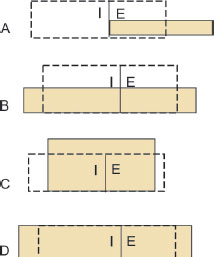38. Which of the diagram best illustrates the fluid shifts resulting from infusion of 1 L of distilled water into the bloodstream?
1. A
2. B
3. C
4. D
39. Which best illustrates the status of a person who received 1 L of a hypertonic saline solution intravenously.
1. A
2. B
3. C
4. D
40. A 60 kg patient has a hematocrit reading of 40 and a plasma volume of 3 liters. What is his total blood volume?
1. 4.0 liters
2. 5.0 liters
3. 6.0 liters
4. 7.0 liters
41. What is the calculated osmolarity of a solution containing 12 mmol NaCl, 4 mmol KCl and 2 millimolar CaCl2 (mOsmol/L)?
1. 16
2. 26
3. 38
4. 32
42. Assuming complete dissociation of all solutes, which of the following solutions would be hyperosmotic to 1 mM NaCl?
1. 1 mM glucose
2. 1.5 mM glucose
3. 1 mM CaCl2
4. 1 mM sucrose
43. Which of the following best describes the changes in cell volume that will occur when red blood cells (previously equilibrated in a 280-mOmol solution of NaCl are placed in a solution of 140 mmol NaCl containing 20 mmol urea, a relatively large but permeant molecule?
1. Cells shrink initially, then swell over time and lyse
2. Cells shrink transiently and return to their original volume over time
3. Cells swell and lyse
4. No change in cell volume will occur
The diagram below illustrates possible changes in RBC volume resulting from a change in ECF composition for a cell equilibrated in 150 mM/L NaCl solution at time 0. Answer questions number 25 and 26 below: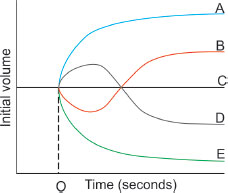44. Which curve best illustrates the volume caused by immersion of the cell into an aqueous solution of 300 mOsm CaCl2?
1. A
2. B
3. C
4. D
45. Which curve best illustrates the volume change caused by immersion of the cell into a mixture of aqueous solution of 200 mOsm/L NaCl and 200 mOsm/L of glycerol?
1. A
2. B
3. C
4. D19
1. Ans. b. 0.4 x body wt.
(Ref: Ganong's Physiology 24th ed. pp 2)
ICF is 40% of total body weight.
2. Ans. d. None
(Ref: Ganong's Physiology 24th ed. pp 2)
ECF is 20% of total body weight, so, its 1/5th of body weigth and 1/3rd of total body water (33%)
3. Ans. c. 28 L
(Ref: Ganong's Physiology 24th ed. pp 2)
ICF is 40% of total body weight. Adult person with 70 Kg body weight, total body water is 42 L and ICF (2/3rd) volume is 28L
4. Ans. a. Na+
(Ref: Ganong's Physiology 24th ed. pp 3)
In ECF Major cation is Na+ and anion is Cl . In ICF major cation is K+ and anion is phosphate.
5. Ans. a. K+
(Ref: Ganong's Physiology 24th ed. pp 2)
Osmolality depends on number of molecules. As K+ concentration is maximum in cell, most osmotically active particle is K+
6. Ans. c. Responsible for Depolarization
(Ref: Ganong's Physiology 24th ed. pp 6)
Donnan effect is distribution of all permeable ions due to presents of non-permeable ions. K+ is responsible for °RMP.
7. Ans. d. 70 ml
(Ref: Human Physiology edited by R.F. Schmidt, G. Thews pp. 643)
Water content of lean body mass is 73.2 ± 3%.
8. Ans. d. Almost 100%
(Ref: Medical Physiology for Undergraduate Students by Indu Khurana, pp 7)
Essentially all of the body K+ is in the exchangeable pool. Only 65–70% of the body Na+ is exchangeable.
9. Ans. d. 30 mm Hg
(Ref: Guyton's Physiology 13th edn. pp. 196)
Plasma proteins cause colloid osmotic pressure or, oncotic pressure. The colloid osmotic pressure of normal human plasma averages about 28 mm Hg; 19 mm of this pressure is caused by molecular effects of the dissolved protein and 9 mm is caused by the Donnan effect—that is, extra osmotic pressure caused by sodium, potassium, and the other cations held in the plasma by the proteins.
10. Ans. c. Mg++
(Ref: Guyton's Physiology 13th edn. pp. 307)
Ma++ is mainly intracellular.
11. Ans. a. 50-100 ml
(Ref: Medical Physiology for Undergraduate Students by Indu Khurana, pp7)
Water loss by the production of sweat from skin can vary from 100 mL/day in routine at room temperature of 23°C to 1400 mL in hot weather to 5000 mL following prolonged exercise.
12. Ans. d. Bone
(Ref: Nutrition Review Volume 19, Issue 5:May 1961 Pages 134–135)
Total sodium content in body is 3500 to 5000 mEq (mMol), which is ~58 mEq/kg body weight. Of this amount, 90% is in the ECF compartment and 10% is in the ICF compartment (primarily in the Bone).20
13. Ans. d. Platelets
(Ref: Clinical Methods: The History, Physical, and Laboratory Examinations. 3rd edition. Chapter 195)
Maximum potassium is in Cell (ICF = 98%), primarily in the muscle, skin, subcutaneous tissue, and red blood cells. As platelet cytoplasm volume is very small as compared to other cell, platelet contains little potassium.
14. Ans. a. 70-80
(Ref: Bulletin of the World Health Organization 2011;89:46-53)
• A person's total blood volume (TBV) is related to body weight. The TBV of a child is around 75–80 ml/kg and is higher in the neonatal period (from 85 ml/kg it rises to a peak of 105 ml/kg by the end of the first month and then drops progressively over ensuing months). Thus, the TBV of a 3.5-kg 2-week-old will be about 350 ml while that of a 10-kg 15-month-old will be about 800 ml.
• Blood volume can be estimated as approximately 70 mL/kg for adults, 80 mL/kg in children and 100 mL/kg in neonates. (Ref: Australian Red cross Society).
15. Ans. (d) High concentration, High molecular weight
(Ref: Boron Text Book 2nd edition page 109)
Plasma proteins concentration in plasma is ∼14 meq/L. However, because these proteins usually have many negative charges per molecule, not many particles (∼1 mM) are necessary to account for these milliequivalents (One equivalent is 1 mol of an ionized substance divided by its valence).
Moreover, even though the protein concentration-measured in terms of grams per liter-may be high, the high molecular weight of the average protein means that the protein concentration-measured in terms of moles per liter (molar concentration)- is very low. Thus, proteins actually contribute only slightly to the total number of osmotically active particles (∼1 mOsm).
16. Ans. b. Low molar concentration, High molecular weight
(Ref. Boron Text book of Medical Physiology 2nd Edn. Page 109)
Note: Options and answer are different here. Explanation is given above.
17. Ans. a. 1½ times the normal
(Ref: Porth Pathophysiology: Concepts of Altered Health States By Charlotte Pooler, pp735)
Edema can be defined by palpable swelling produced by expansion of interstitial fluid volume. Edema does not become clinically evidence until the interstitial fluid volume has been increased by 2.5L to 3.5L. So, if we consider the normal ISF is around 10L, best possible answer of the question will be 1.5 times than normal.
18. Ans. b. 17mmHg
(Ref: Guyton's Physiology 13th edn. pp. 318)
Three major safety factors prevent exce-ssive fluid accumulation in the interstitial spaces:
• Low compliance of the interstitium in the negative pressure range = 3 mm Hg
• Safety factor caused by increased lymph flow = 7 mm Hg.
• Safety factor caused by washdown of proteins from the interstitial spaces = 7 mm Hg.
Therefore, the total safety factor against edema is about 17 mm Hg. is means that the capillary pressure in a peripheral tissue could theoretically rise by 17 mm Hg, or approximately double the normal value, before marked edema would occur.21
(Ref: Guyton-Physiology-13th edn.pp 309)
Here, you need two substances: one for ECF estimation and one for plasma volume estimation. ISF = (ECF-Plasma). ECF is measured by Inulin (Best), 22Na, 125I-iothalamate, thiosulfate, mannitol, sucrose. Plasma is measured by 125I-albumin, Evans blue dye (T-1824).
So, obviously D is the answer of choice.
20. Ans. a. Most of the K+ are intracellular, c. An essential electrolyte for different organ functions, d. Actively secreted in the distal tubules
(Ref: Clinical Methods: The History, Physical, and Laboratory Examinations. 3rd edition. Chapter 195)
• Total body potassium is approximately 55mEq/kg body weight. Of this amount, 98% is in the intracellular compartment (primarily in the muscle, skin, subcutaneous tissue, and red blood cells) and 2% is in the extracellular compartment.
• Some active potassium secretion takes place in the most distal part of the distal convoluted tubules but potassium excretion is primarily a passive process.
• Na-K-ATPase pump maintains the distribution of K+ ion.
21. Ans. a. Cell
(Ref: Clinical Methods: The History, Physical, and Laboratory Examinations. 3rd edition. Chapter 195)
Maximum potassium is in Cell (ICF = 98%), primarily in the muscle, skin, subcutaneous tissue, and red blood cells. Maximum sodium stored in bone.
22. Ans. b. 58
(Ref: Nutrition Review Volume 19, Issue 5:May 1961 Pages 134–135)
Total sodium content in body is 3500 to 5000 mEq (mMol), which is ~58 mEq/kg body weight. Of this amount, 90% is in the ECF compartment and 10% is in the ICF compartment (primarily in the Bone).
23. Ans. a. 0.4%
(Ref: Clinical Methods: The History, Physical, and Laboratory Examinations. 3rd edition. Chapter 195)
Total potassium content of body 3000 to 3750 mEq (mMol), which is ~53 mEq/kg body weight. Of this amount, 98% is in the ICF compartment (primarily in the muscle) and 2% is in the ECF compartment. Because plasma constitutes 1/4th of ECF, K+ content of plasma will be 0.5% of total body K+.
24. Ans. C. 40-45L
(Ref: Ganong's Physiology 25th ed. Pp 4)
A man weighing 70kg, total water content of body is 42L (60%). ECF = 14L and ICF = 28L
25. Ans. c. 285-295 mOsm/L
(Ref: Guyton-Physiology-13th edn.pp 309)
26. Ans. a. Water constitutes 60% of the body weight
(Ref: Ganong's Physiology 24th ed. pp 2)
c. ECF volume can be determined by dilution methods
Plasma volume is 5% of total body weight. In a 70 kg person, plasma volume is 3.5 L and total body water is 42 L (60% of body weight). So, plasma is 8.3% of total body water [(3.5/42)×100]
27. Ans. b. 25%
(Ref: Ganong's Physiology 24th ed. pp 2)
ECF is 20% of total body weight. So, close answer is 25% with the provided options.22
28. Ans. a. Total body water constitutes about 60% of body weight
b. Most intracellular water is the musculoskeletal system
e. Extracellular fluid can be measured by dilution method
(Ref: Ganong's Physiology 24th ed. pp 2)
ECF constitute 20% of total body weight. Total plasma is 5% of body weight.
29. Ans. d. I125 albumin for blood volume
(Ref: Guyton-Physiology-13th edn.pp 49)
I125 albumin is used for plasma volume not blood volume.
30. Ans. c. Inulin
(Ref: Guyton-Physiology-13th edn.pp 49)
Inulin is best for ECF measurement.
31. Ans. a. Na+ c. Cl d. HCO3
(Ref: Ganong's Physiology 24th ed. Pp 3)
Predominant ECF: Na+, Cl, HCO3 and Ca++
Predominant ICF: K+, Mg++, phosphate and protein.
32. Ans. b. Phosphorus and Mg++ are major ions intracellularly,
c. Na+, Cl principal ions in ECF
d. Kidney tightly regulates Na+, K+, Cl composition
(Ref: Ganong's Physiology 24th ed. pp 3)
33. Ans. a. Normal serum level is 135-145 mEq/L
b. Daily intake is 150 mmol of NaCl
c. Major portion is extracellular
(Ref: Ganong's Physiology 24th ed. pp 3)
Major reserve of Na is bones not muscle.
34. Ans. c. 10-18 years
(Ref: Fluid and Electrolyte Balance By Norma M. Metheny)
Difference is not seen upto the age of puberty
35. Ans. b. 22.4 atm
(Ref: Guyton's Physiology 12th edn.pp. 299 & 13th edn.pp 51)
“At 37°C, a concentration of 1 osmole per liter will cause 19,300 mm Hg osmotic pressure in the solution. Likewise, 1 mOsmol per liter concentration is equivalent to 19.3 mm Hg osmotic pressure.”
36. Ans. c. Urea
(Ref: Guyton's Physiology 12th edn. pp. 299 & 13th edn.pp 52)
“Highly permeating substances, such as urea, can cause transient shifts in fluid volume between the ICF and ECF, but given enough time, the concentrations of these substances eventually become equal in the two compartments and have little effect on intracellular osmolality and volume under steady-state conditions.” That's why urea is an ineffective osmoles.
37. Ans. c. 375 ml
Water is a hypotonic fluid so, it will be distributed equally in both ECF and ICF according to their volume.
So,
• 2/3rd of 1500 ml will be distributed in ICF = 1000 ml
• 1/3rd of 1500 ml will be distributed in ECF = 500 ml
• ISF is 3/4th of ECF = 3/4th of 500 ml = 375 ml.
38. Ans. b. B
Infusion of distilled water would decrease body osmolarity and increase the volume of the ECF. The decreased ECF osmolarity would cause water to diffuse into cells and raise ICF volume.
39. Ans. None
Infusing hypertonic saline will increase body osmolarity. Since fluid is given, the volume 23of the ECF compartment will increase. The increased osmolarity of the ECF will cause water to diffuse out of cells, decreasing ICF volume. None of the diagram is matching.
40. Ans. b. 5.0 Liters
Hematocrit (Hct) = 40% = 0.4
Blood volume = Plasma volume/(1–Hct) = 3/(1–0.4) = 5 L
41. Ans. c. 38
1 mmol solution has 1mOsmol when the solute does not dissociate. NaCl and KCl both dissociate into two molecules and CaCl2 dissociae into 3 molecules. So, 12 mmol of NaCl has an osmolality of 24 mOsmol, 4 mmol of KCl = 8 mOsmol and 2 mmol of CaCl2 = 6 mOsmol. Total 38 mOsmol.
42. Ans. c. 1 mM CaCl2
The 1 mM CaCl2 solution (osmolarity = 3 mOsm/L) is hyperosmotic to 1 mM NaCl (osmolarity = 2 mOsm/L). The 1 mM glucose, 1.5 mM glucose, and 1 mM sucrose solutions are hyposmotic to 1 mM NaCl.
43. Ans. b. Cells shrink transiently and return to their original volume over time
Cell is placed in a solution of 140 mmol NaCl with 20-mmol urea. A solution of 140 mmol NaCl has an osmolarity of 280 mOsmol, which is iso-osmotic relative to “normal” intracellular osmolarity. If RBC were placed in 140 mmol NaCl alone, there would be no change in cell volume. But the additional presence of 20-mmol urea, however, increases the solution's osmolarity and makes it hypertonic relative to the ICF solution. Water will initially move out of the cell, but because the plasma membrane is permeable to urea, urea will diffuse into the cell and equilibrate across the plasma membrane. As a result, water will re-enter the cell, and the cell will return to its original volume. So, B better answer>>D.
44. Ans. c. C
• The RBC was equilibrated in 150 mM NaCl (means 300 mOsmol NaCl) that is an isotonic saline solution. A 300 mOsm CaCl2 solution is also an isotonic solution of a nonpenetrating solute, thus, there will be no change in RBC volume.
45. Ans. b. B
• 200 mOsm NaCl is nonpenetrating but the 200 mOsm glycerol penetrates slowly. Initially the effective osmolarity of the solution is 400 mOsm (200 NaCl+ 200 glycerol), thus the cell shrinks. With time the glycerol penetrates and the final effective osmolarity of the solution is only 200 mOsm due to the NaCl. Thus, as the glycerol penetrates, water moves into the red blood cell and final volume will be greater than at time zero. Curve B, which shows an initial shrinkage then overall swelling, is the best answer.